# How do you write an integral(∫) in LaTeX?

Default \int is the popular command by which the integral symbol is represented in the document. And the bigints package is designed to enlarge the shape of the symbol one by one.

\documentclass{article}
\usepackage{bigints}
\begin{document}
$\int f(x) dx$
$\int \frac{\tan^{-1}x}{x(x^2+1)}dx$
%Use bigints package
$\bigintssss f(x)dx ,\bigintsss f'(x)dx ,\bigintss f''(x)dx,\bigints \frac{f(x)}{g(x)}dx,\bigint \frac{f'(x)}{g'(x)}dx$
\end{document}

Output :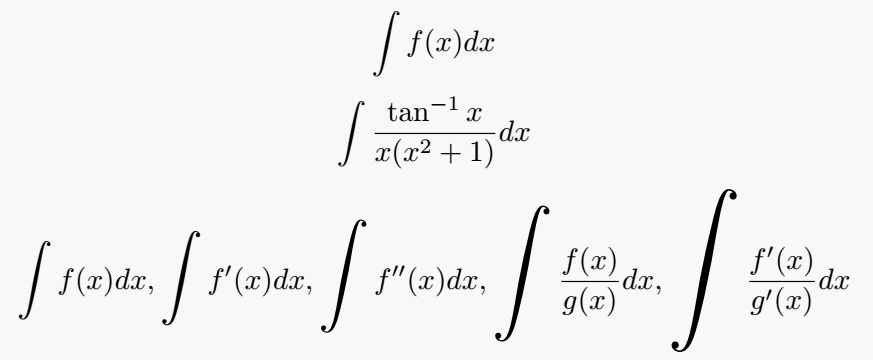## Integral symbol with above and below limits

You can use limits on integral symbols in two ways. First, it uses superscript and subscript with the \int command, so that the value of limit will sit lightly on the right side with a symbol.

Second, to use the limits above and below the integral symbol, you need to use \limits command along with \int command.

\documentclass{article}
\begin{document}
$\int_5^7 \frac{x^3}{x^2-4}dx$
$\int\limits_5^7 \frac{x^3}{x^2-4}dx$
$\int_0^\infty \frac{\sin x}{x(1+x^2)}dx$
$\int\limits_0^\infty \frac{\sin x}{x(1+x^2)}dx$
\end{document}

Output :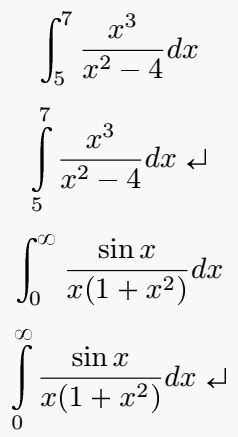Of course, you can see some differences by looking at the output above.

Instead of using so many commands, you can convert them to one command with the help of \newcommand.

\documentclass{article}
\usepackage{bigints}
\newcommand{\integral}{\int\limits_{#1}^{#2}}
\begin{document}
$\integral{5}{7} \frac{x^3}{x^2-4}dx$
$\integral{0}{\infty}\frac{\sin x}{x(1+x^2)}dx$
$\integral{X_1}{X_2}a_i x^i dx= a_i \frac{X^{i+1}_2 - X^{i+1}_1}{i+1}$
\end{document}

Output :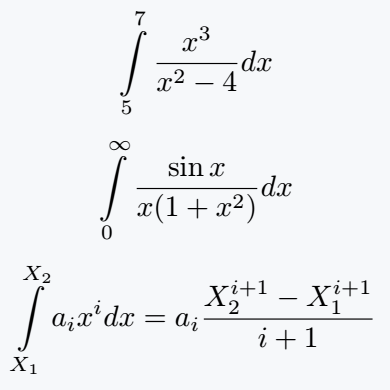## Double integral symbol [Surface integral]

When you use limits with double integral, need to use the value of limit twice with \int command.

\documentclass{article}
\usepackage{amsmath}
\newcommand{\integral}{\int\limits^{#1}_{#2}}
\begin{document}
$\iint_S \textbf{F}.d\textbf{S}= \iint\limits_S \textbf{F.n}dS$
$\integral{2\pi}{0}\integral{1}{0} \;r3\; \sin 2\theta\; dr \;d\theta$
$\integral{2\pi}{v-0}\integral{1}{n-0}\langle u\sin v,1-u^2,-u\cos v\rangle.\langle 2u^2\cos v,2u^2 \sin v,u\rangle dudv$
\end{document}

Output :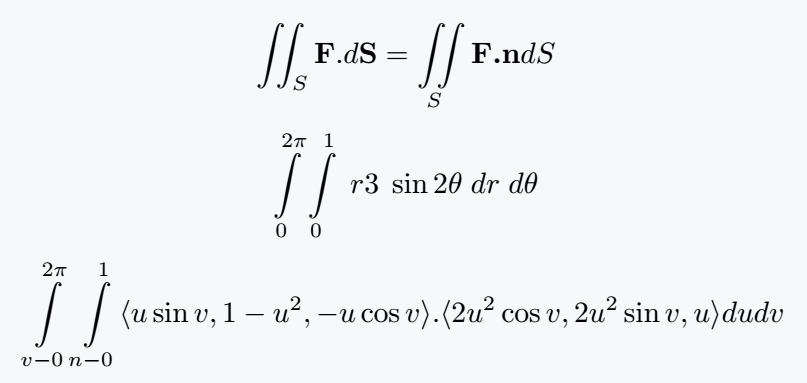If you think, you will use a down limit then using \iint for double integral is enough.

## Triple integral symbol

In this case, you need to represent it in the same way as shown above. However, the triple integral requires the use of the \iiint command.

\documentclass{article}
\usepackage{amsmath}
\newcommand{\integral}{\int\limits^{#1}_{#2}}
\begin{document}
$\iiint_V \nabla \cdot\textbf{F}dV$
$V =\iiint_S dx\; dy\; dz\;$
$\integral{2}{0}\integral{4}{x^2}\integral{2-x}{0}\; f(x,y,z)\;dz\;dy\;dx$
\end{document}

Output :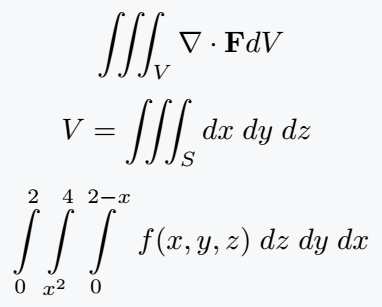## Closed integral symbol

Pxfonts package has been implemented for Closed Integral which contains all types of commands. The same symbol is also present in other packages like stix, mathabx, etc.

\documentclass{article}
\usepackage{pxfonts}
\begin{document}
$\oint,\ointclockwise,\ointctrclockwise$
$\oiint,\oiintclockwise,\oiintctrclockwise$
$\oiiint,\oiiintclockwise,\oiiintctrclockwise$
$\sqint,\sqiint,\sqiiint$
\end{document}

Output :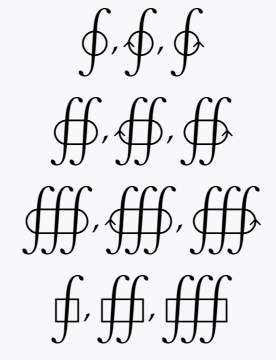## Integral evaluation bar

Integration evolution bar can be defined manually. Again, you can solve the \eval command from the physics package. Following code solves the problem in two ways.

\documentclass{article}
\usepackage{physics}
\begin{document}
$\frac{x^2}{2} \bigg|_{a_1}^{a_2}$
%Use physics package
$\eval{x}_0^\infty$
\end{document}

Output :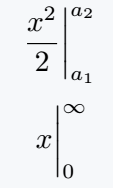## Square brackets with a limit

In this case, the square bracket must be larger than the expression. For this manually the \bigg command has been used. With this, you can pass the value in superscript and subscript to give a limit.

\documentclass{article}
\usepackage{physics}
\begin{document}
$\bigg[\frac{x^2}{2} \bigg]_{a_1}^{a_2}$
$\bqty\bigg{x}_{-\infty}^{+\infty}$
\end{document}

Output :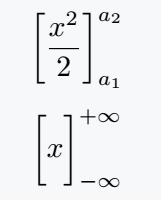#### Md Jidan Mondal

LaTeX expert with over 10 years of experience in document preparation and typesetting. Specializes in creating professional documents, reports, and presentations using LaTeX.